Search

About 20 Search Results Matching Types of Worksheet, Worksheet Section, Generator, Generator Section, Subjects matching Geometry, Grades matching 4th Grade, Similar to Valentine's Day Worksheet - Counting Eighteen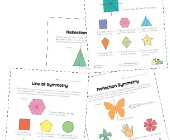Symmetry Worksheets

Help kids learn about the concept of symmetry with...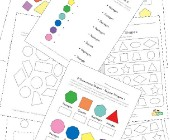Geometry Worksheets

Help kids learn the early basics of geometry inclu...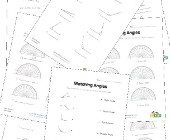Angles Worksheets

Help kids learn about angles with this collection ...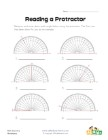Reading a Protractor Worksheet 2

Read each protractor and write down the angles the...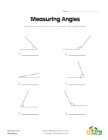Measuring Angles Worksheet 1

Measure each of the angles with a protractor and w...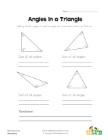Angles in a Triangle Worksheet

Kids learn that the angles of a triangle always ad...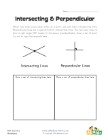Intersecting and Perpendicular Lines Worksheet

Learn about intersecting and perpendicular lines.